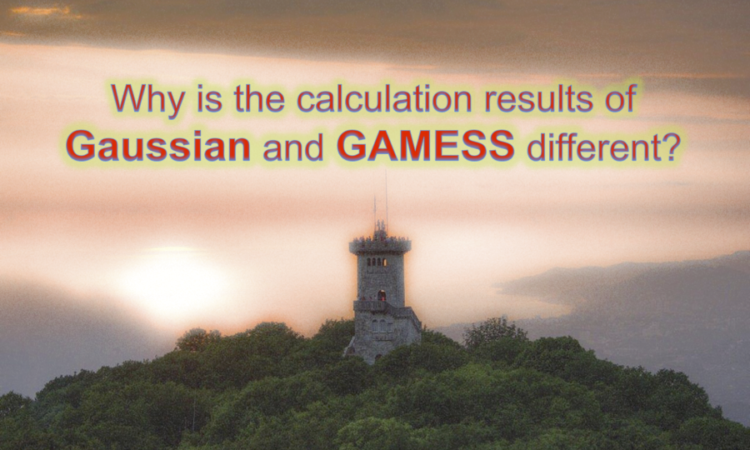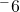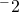# Why is the calculation results of Gaussian and GAMESS different?

I’ve seen the comments that the calculation result by Gaussian is not exactly the same as the that of GAMESS. Even if you use the same calculation software, it may not exactly match reference data such as paper or database. Why is the calculated result inconsistent despite calculation with the same theory/basis set?

#### It is normal that results are different depending on calculation software!?

Originally, if the same molecule is calculated under the same condition, the same result should be obtained. However, in reality, it is not necessarily consistent. That is because the specifications set by default of the QM calculation software are different.

For example, the threshold of computation termination set by SCF calculation and structure optimization. The threshold of the energy gradient used for the convergence criteria in the structure search of GAMESS and Gaussian is as follows.
GAMESS(US)： 1.0D-4 hartree/bohr
Gaussian(g03)： 4.5D-4 hartree/bohr

As GAMESS and Gaussian use different VWN functional, if you use B3LYP as default setting, you would get the different calculation result.
GAMESS(US)： VWN5
Gaussian(g03)： VWN3(VWN1RPA)

If you want to use VWN3 in GAMESS(US) instead of using VWN5 that is used in Gaussian, please specify the keyword “DFTTYP=B3LYPV3”. In the case of Firefly(PC GAMESS), VWN5 is the default setting(B3LYP=NWCHE)

There are some keyword that you can change as described above, however, there are also some setting that you can’t change. For example, the cutoff method and convergence routine of each calculation.
For reference data such as scientific papers, almost no one mention about these detailed settings, so we face the problem that calculation does not completely match even if we use the same level of theory and the basis set.

#### What if the results are different though using the same calculation software?

So, what is the reason why the calculation results do not perfectly match even when using the same program? There are two main reasons for this.

1) Input file creation mistake
2) Program version difference

Also, I would like you to remember that the calculation error is also caused by the difference in the initial structure(value) of the molecule. This is because errors may occur due to numerical processing during the process (rounding up, truncation, etc.). For example, it is common to generate an error of about 10Hartree in energy value, about 10Å in coupling distance and about 10degrees in bonding angle.

Another thing to note is that the same program also has different versions. This is because there is a possibility that the default setting value has been changed when updating even the same program, like gaussian09 and gaussian16. Based on this fact, it is also important to clearly state the version of which calculation program you used .

#### Summary

In this article, we explained several reasons why the calculation result does not match the reference data. In particular, I often hear the mistake that the calculation result does not match without knowing that the VWN functional of the B3LYP function is different in default setting between GAMESS and Gaussian. When doing calculations between different programs, it is also important to always read the manuals on what kind of differences are present in default calculation methods and so on.

#### Related Article

This site uses Akismet to reduce spam. Learn how your comment data is processed.